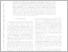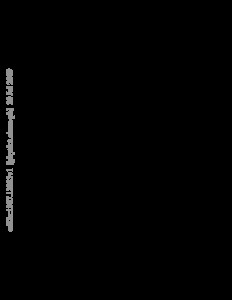Repository: Freie Universität Berlin, Math Department

# Non-Markovian barrier crossing with two-time-scale memory is dominated by the faster memory component

Kappler, Julian and Hinrichsen, Victor B. and Netz, Roland R. (2019) Non-Markovian barrier crossing with two-time-scale memory is dominated by the faster memory component. The European Physical Journal E, 42 (119). ISSN 1292-8941Preview

3MB

## Abstract

We investigate non-Markovian barrier-crossing kinetics of a massive particle in one dimension in the presence of a memory function that is the sum of two exponentials with different memory times τ 1 and τ 2 . Our Langevin simulations for the special case where both exponentials contribute equally to the total friction show that the barrier crossing time becomes independent of the longer memory time if at least one of the two memory times is larger than the intrinsic diffusion time. When we associate memory effects with coupled degrees of freedom that are orthogonal to a one-dimensional reaction coordinate, this counterintuitive result shows that the faster orthogonal degrees of freedom dominate barrier-crossing kinetics in the non-Markovian limit and that the slower orthogonal degrees become negligible, quite contrary to the standard time-scale separation assumption and with important consequences for the proper setup of coarse-graining procedures in the non-Markovian case. By asymptotic matching and symmetry arguments, we construct a crossover formula for the barrier crossing time that is valid for general multi-exponential memory kernels. This formula can be used to estimate barrier-crossing times for general memory functions for high friction, i.e. in the overdamped regime, as well as for low friction, i.e. in the inertial regime. Typical examples where our results are important include protein folding in the high-friction limit and chemical reactions such as proton-transfer reactions in the low-friction limit.

Item Type: Article arXiv.org > physics > arXiv:1907.12883 Mathematical and Computer Sciences > Mathematics > Applied Mathematics Department of Mathematics and Computer Science > Institute of Mathematics 2402 Monika Drueck 18 Feb 2020 10:56 20 Feb 2020 10:43

Repository Staff Only: item control page